Email us to get an instant 20% discount on highly effective K-12 Math & English kwizNET Programs!

#### Online Quiz (WorksheetABCD)

Questions Per Quiz = 2 4 6 8 10

### MEAP Preparation - Grade 4 Mathematics1.41 Types of Fractions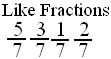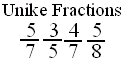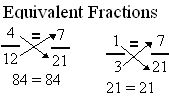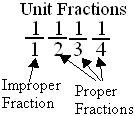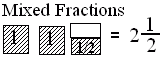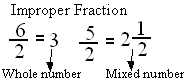Directions: Answer the following questions. Also write at least 5 examples of each type of fractions.
 Q 1: If the numerator is more than the denominator in a fraction, the fraction is called a _________.proper fractionimproper fractionmixed fraction Q 2: When a products obtained by cross multiplication give the same number, then the fractions are ____(or equal).mixedequivalentproper Question 3: This question is available to subscribers only! Question 4: This question is available to subscribers only!